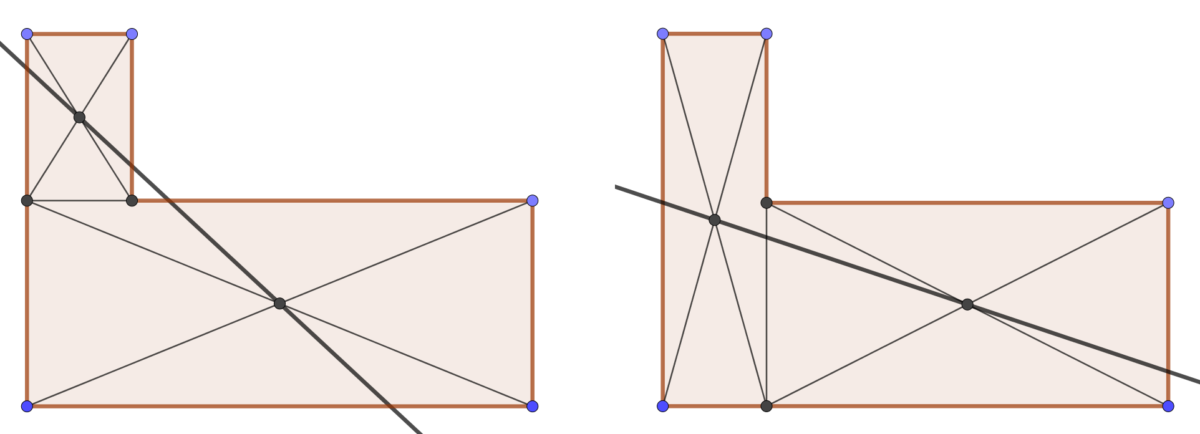# L-bisectorThis post is about a geometry Riddler puzzle involving bisecting a shape using only a straightedge and a pencil. Here is the problem:

Say you have an “L” shape formed by two rectangles touching each other. These two rectangles could have any dimensions and they don’t have to be equal to each other in any way. (A few examples are shown below.)

Using only a straightedge and a pencil (no rulers, protractors or compasses), how can you draw a single straight line that cuts the L into two halves of exactly equal area, no matter what the dimensions of the L are? You can draw as many lines as you want to get to the solution, but the bisector itself can only be one single straight line.Here is my solution:
[Show Solution]

## 7 thoughts on “L-bisector”

1.Jason Weisman says:

Laurent, possibly a small point but important for the solution to the problem as stated. Your first solution above does not necessarily cut the L in two parts which is required by the puzzle statement. See top, left figure indicating the L cut into three parts.

I think the best general approach is the “alternative solution.” Drawing the line between centers of the large rectangle and virtual rectangular space will guarantee this line segment cuts the L in two halves.

1.Laurent says:

That’s a good point. I wasn’t thinking about that at all — I interpreted “two halves” as meaning what’s on one side of the line vs the other. If you want each “half” to be a connected shape then you’re right; using the alternative solution is the only solution I presented that also guarantees you’ll get two connected halves. Thanks for the comment!

2.RQ says:

There is an infinite number of ways to split a shape into two equal areas. The two bisecting lines you found for the L-shape intersect at an interesting point. Any line through this point will also bisect the L.

1.Laurent says:

In fact, all three bisecting lines I found for the L-shape intersect in the same point! However, this point does not generate an infinite family of bisectors. The three bisectors I found are the only bisectors that pass through this point. While it’s true that there are infinitely many lines that bisect the L-shape, there aren’t infinitely many that pass through this point!

2.Jason Weisman says:

“There are an infinite number of ways to split an L shape into two equal areas.” This is a true statement. Take a line segment with any angle between 0 and 90 degrees with respect to the horizontal, move it from left to right across the L. There must be a position at which half of the L is on each side of the line. However, it is not possible to determine this position and draw this line segment using only a straight edge and pencil.

“Any line through the point determined using the steps described in the puzzle solution will also bisect the L.” This is not a true statement for an asymmetrical L shape.

3.Jack Carey says:

Is there any method to prove that it 100% fully cut the L in half?

1.Laurent says:

I presented several methods for cutting the L in half (exactly). Could you clarify what you mean by “fully cut the L in half”?